# Normal Distribution and Normality

The normal distribution is also known as a Gaussian distribution. It is the most frequently referenced distribution and it approximates many natural tendencies of data. The normal distribution is a probability distribution of a continuous random variable whose values spread symmetrically around the mean. A normal distribution can be completely described by using its mean (μ) and variance (σ2), because mean and variance determines the shape of the distribution. When a variable x is normally distributed, we denote x ~ N(μ, σ2).

The probability density function of the normal distribution is: `f(x) = (1/(sqrt(2Pisigma^2))) e^(-((x-mu)^2)/(2sigma^2))`

# Characteristics of the Normal Distribution

## Shape of Normal Distribution

• The probability density function curve of a normal distribution is “bell” shaped.
• All normal distributions are symmetric and have bell-shaped density curves with a single peak.
• Location of Normal Distribution.
• If a data sample or population is normally distributed, the mean, median and the mode will have the same approximate values.
• The probability density curve of the normal distribution is symmetric around a center value which is the mean, median and mode.
• The spread or variation of normally distributed data can be described using variance or standard deviation.
• The smaller the variance or standard deviation, the less variability in the data set.
• 68-95-99.7 Rule.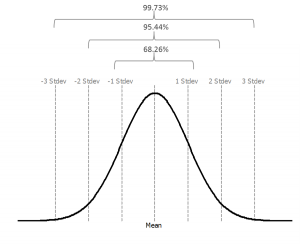The 68-95-99.7 rule or the empirical rule in statistics states that for a normal distribution.

• About 68% of the data fall within one standard deviation of the mean, that is, between μ-σ and μ+σ.
• About 95% of the data fall within two standard deviations of the mean, that is, between μ-2σ and μ+2σ.
• About 99.7% of the data fall within three standard deviations of the mean, that is, between μ-3σ and μ+3σ.
• The image below depicts this rule:

# Normality

Not all distributions with a “bell” shape are normal distributions, so we need to check whether the data are normally distributed. To do so, we should run a normality test. There are different normality tests available.

• Anderson-Darling test
• Sharpiro-Wilk test
• Jarque-Bera test
• Normality Testing

Normality tests are used to determine whether the population of interest is normally distributed. As discussed above there are several normality tests available like Anderson-Darling test, Sharpiro-Wilk test, Jarque-Bera test and so on. For any of these tests, the null and alternative hypothesis are generally the same:

Null Hypothesis (H0): The data are normally distributed.
Alternative Hypothesis (Ha): The data are not normally distributed.

# Use Minitab to Run a Normality Test

Steps to run a normality test in Minitab. (Open Sample Data.xlsx and use the “One Sample T-Test” tab)

• Click Stat -> Basic Statistics -> Normality Test.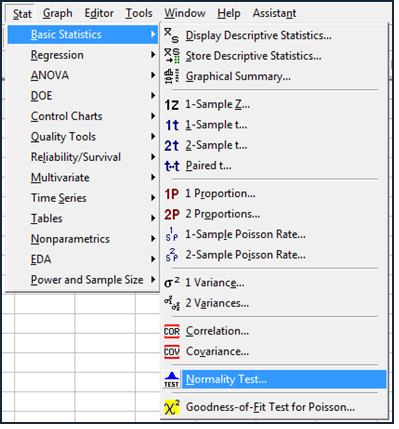• A new window named “Normality Test” pops up.
• Select “Data column” as the “Variable”.
• Click “OK”.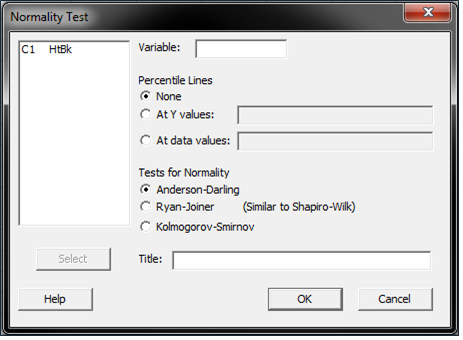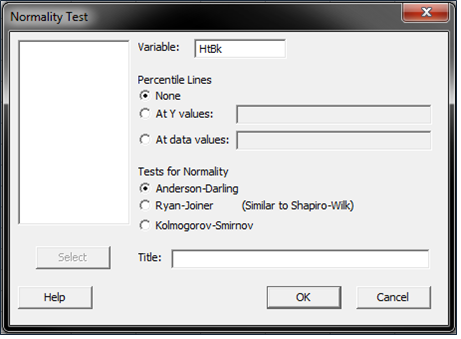• The normality test results appear in the new window.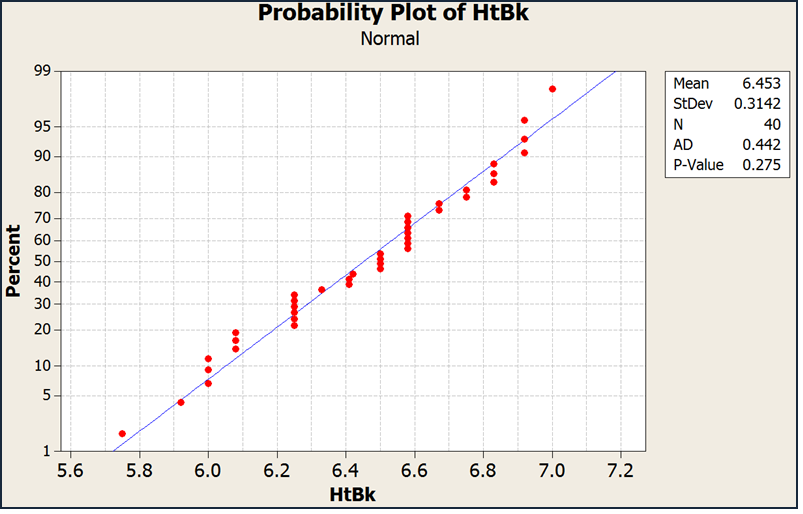Conclusion: Check the p-value in the graph

Remember our assumptions?

• Null Hypothesis (H0): The data are normally distributed.
• Alternative Hypothesis (Ha): The data are not normally distributed.
• If the p-value is greater than the alpha level (0.05), we fail to reject the null hypothesis and claim that the data are normally distributed.
• If the p-value is less than the alpha level (0.05), we reject the null hypothesis and claim that the data are not normally distributed.